# Question 4 (25% total) Use the Duhamel Integral method to determine expression for the response x(t) of an undamped SDO...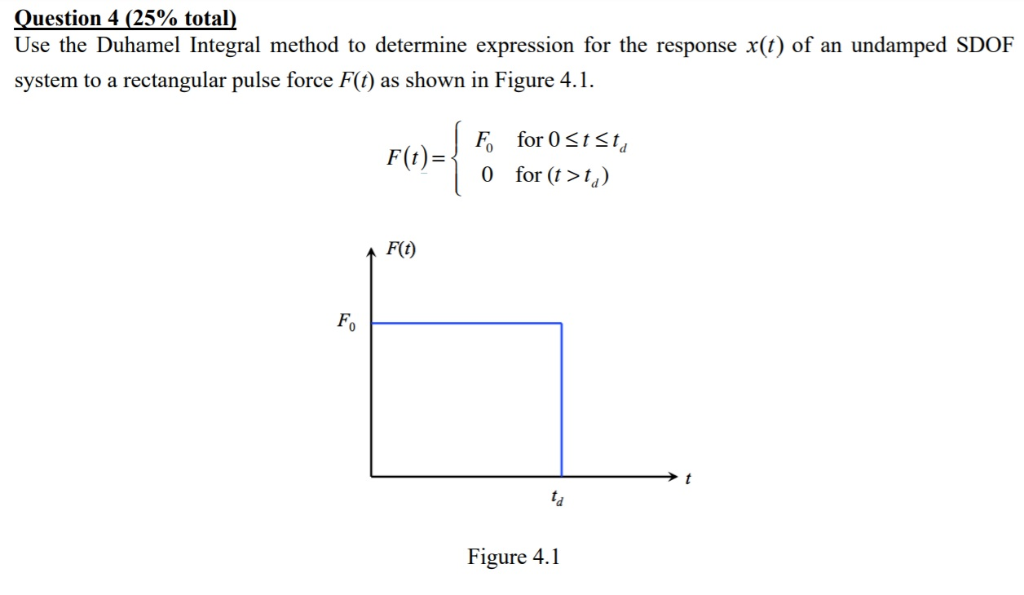Question 4 (25% total) Use the Duhamel Integral method to determine expression for the response x(t) of an undamped SDOF system to a rectangular pulse force F(t) as shown in Figure 4.1 F for O StSt Ffor («>) F(t) Fo Figure 4.1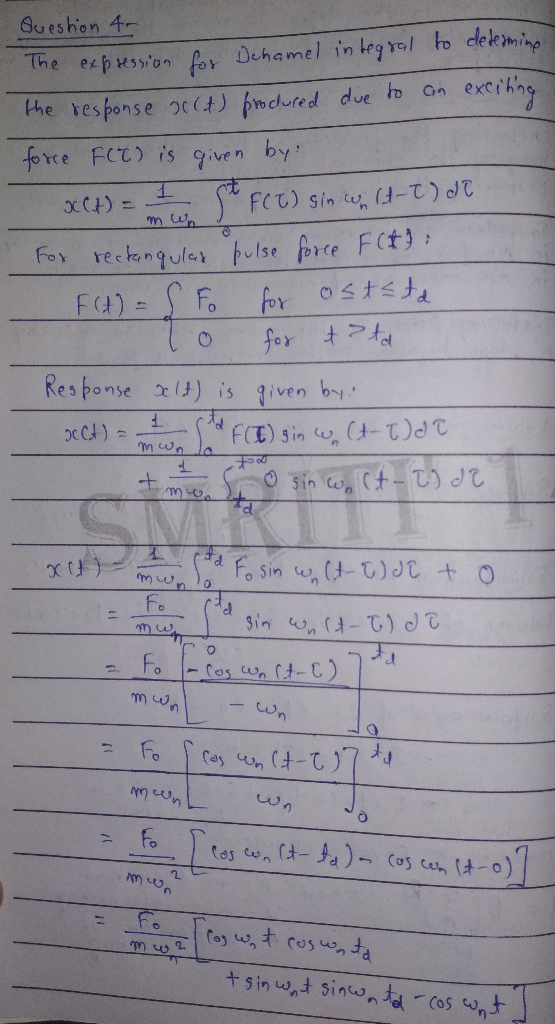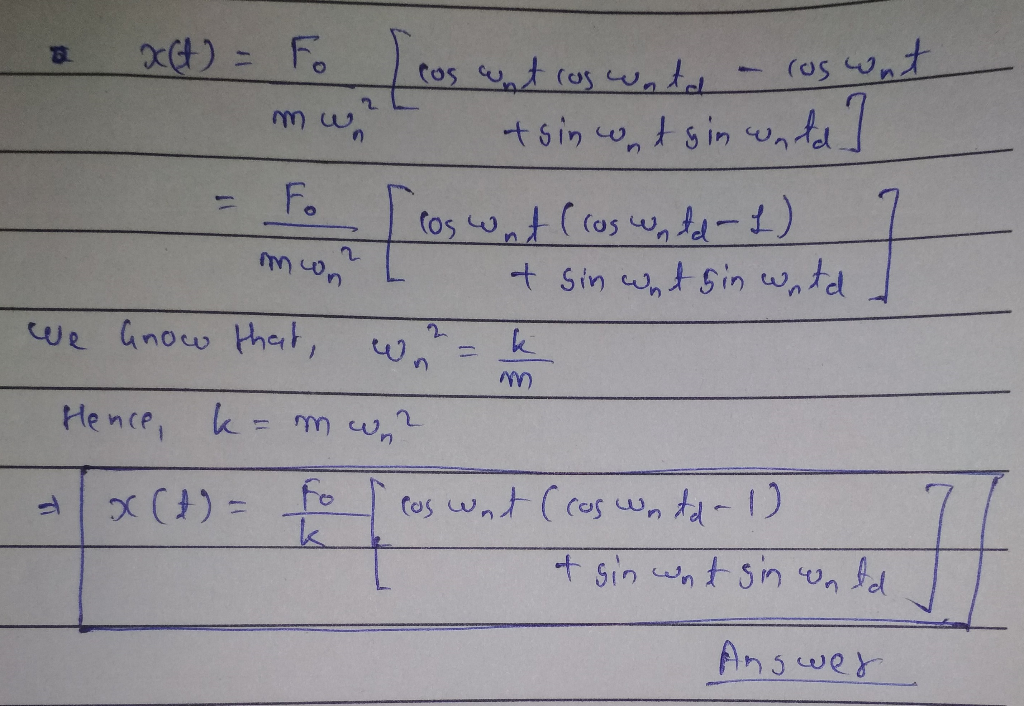##### Add Answer of: Question 4 (25% total) Use the Duhamel Integral method to determine expression for the response x(t) of an undamped SDO...
Similar Homework Help Questions
• ### 2. (25%) Consider an undamped system subject to a rectangular pulse given by F(t) Fo for...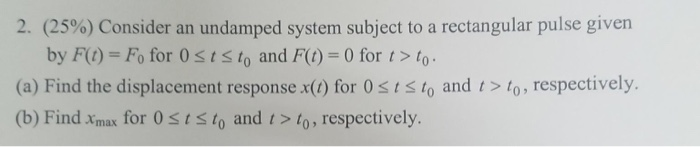2. (25%) Consider an undamped system subject to a rectangular pulse given by F(t) Fo for 0sts to and Ft) = 0 for t > to (a) Find the displacement response x() for 0ststo and t>to, respectively. (b) Find xmax for 0ststo and t> to, respectively. 2. (25%) Consider an undamped system subject to a rectangular pulse given by F(t) Fo for 0sts to and Ft) = 0 for t > to (a) Find the displacement response x() for 0ststo...

• ### Question Four (a) Determine the response x() for the undamped system subjected to the force F...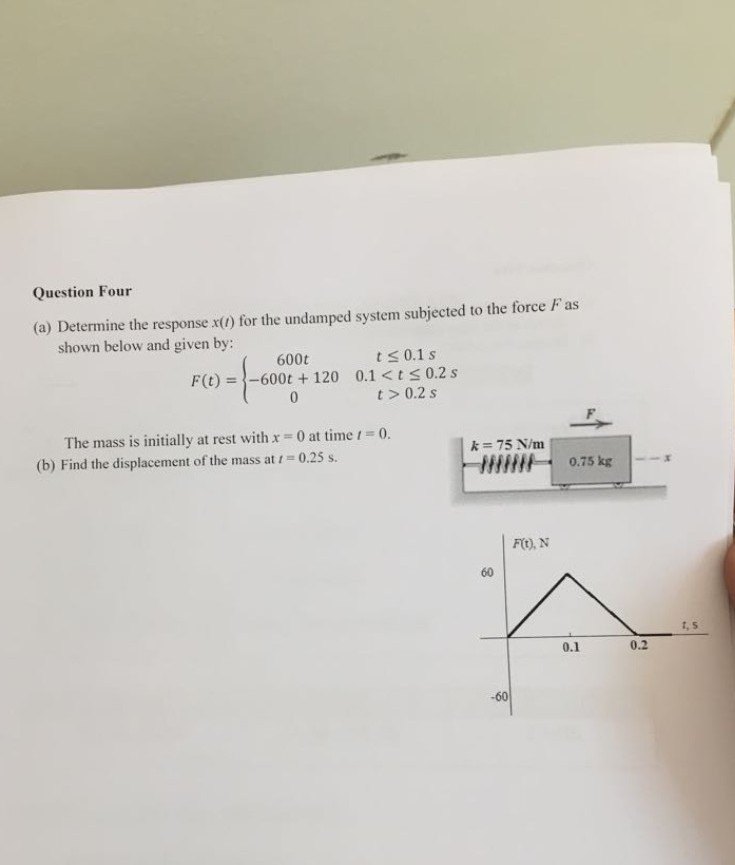Question Four (a) Determine the response x() for the undamped system subjected to the force F as shown below and given by: ts 0.1s F(t) =-600t +120 0.1 <t s 0.2 s t> 0.2s 600t 0 The mass is initially at rest with x 0 at time 1 0. (b) Find the displacement of the mass at 1 0.25 s. k 75 N/m 0.75 kg F), N 1, S 0.2 0.1 Question Four (a) Determine the response x() for the...

• ### State vIIU F(0) 3) (25 pts.) Find the response of an undamped single degree of freedom systerm to the excitation F(t...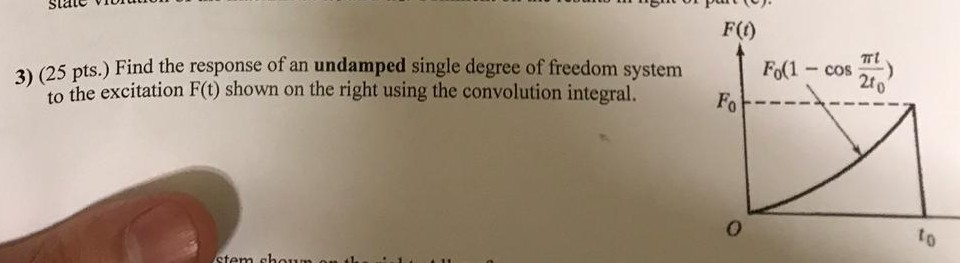State vIIU F(0) 3) (25 pts.) Find the response of an undamped single degree of freedom systerm to the excitation F(t) shown on the right using the convolution integral. cos 21 to State vIIU F(0) 3) (25 pts.) Find the response of an undamped single degree of freedom systerm to the excitation F(t) shown on the right using the convolution integral. cos 21 to

• ### A reciprocating pump weighing W-150 lb, is mounted at a middle of a steel plate of thickness 0.5 ...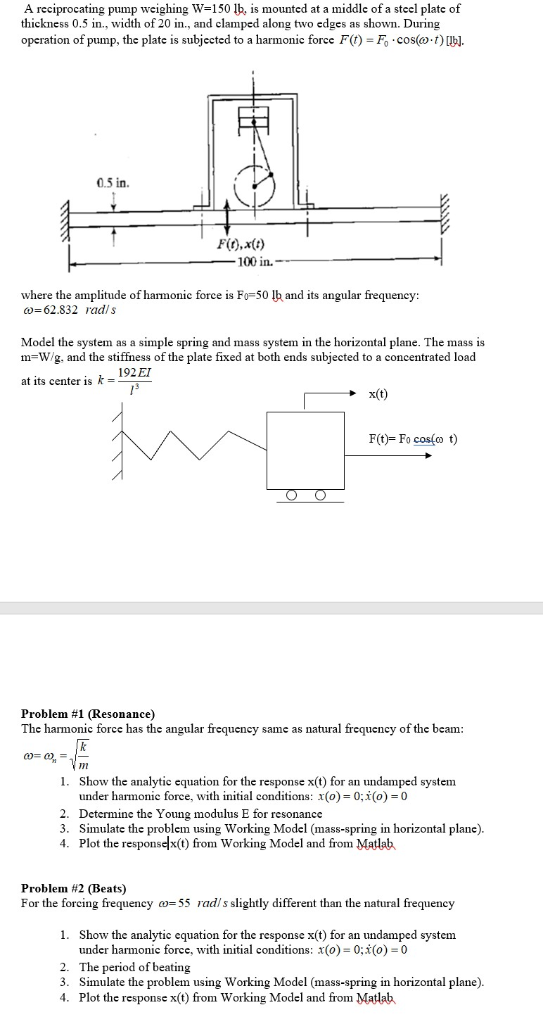A reciprocating pump weighing W-150 lb, is mounted at a middle of a steel plate of thickness 0.5 in., width of 20 in., and clamped along two edges as shown. During operation of pump, the plate is subjected to a harmonic force Ft)-Fo.t) ibl. 0.5 in. Fio),x(t) 100 in. where the amplitude of harmonic force is F俨50 lh and its angular frequency: 62.832 radls Model the system as a simple spring and mass system in the horizontal plane. The mass...

• ### uestion 2 (25% total a) For a lightly-damped SDOF system, let x, and 1,- be the free vibration displacement amplitudes...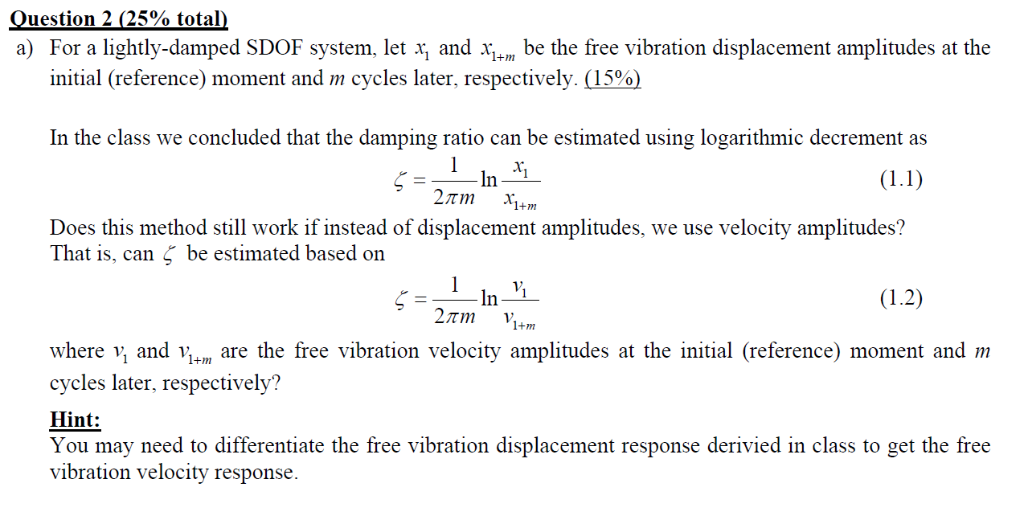uestion 2 (25% total a) For a lightly-damped SDOF system, let x, and 1,- be the free vibration displacement amplitudes at the initial (reference) moment and m cycles later, respectively. (15%) In the class we concluded that the damping ratio can be estimated using logarithmic decrement as (LI) 27m Does this method still work if instead of displacement amplitudes, we use velocity amplitudes? That is, can be estimated based on 1+m where v, and Vi+ are the free vibration velocity...

• ### Question 3) Given a system with an impulse response of: h(t) = te-2t(U(t)-U(t-4)). Use MATLAB to ...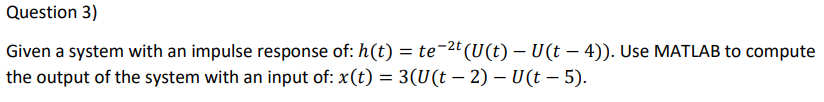Question 3) Given a system with an impulse response of: h(t) = te-2t(U(t)-U(t-4)). Use MATLAB to compute the output of the system with an input of: x(t)-3(U(t -2)-U(t - 5) Question 3) Given a system with an impulse response of: h(t) = te-2t(U(t)-U(t-4)). Use MATLAB to compute the output of the system with an input of: x(t)-3(U(t -2)-U(t - 5)

• ### Question 3 (35 marks) Consider a mechanical system shown in Figure 3. The system is at rest for t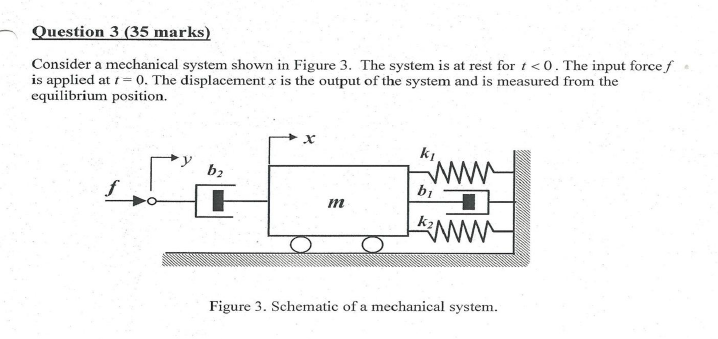Question 3 (35 marks) Consider a mechanical system shown in Figure 3. The system is at rest for t<0. The input force f is applied at 0. The displacement x is the output of the system and is measured from the equilibrium position. kI b2 bi it Figure 3. Schematic of a mechanical system. (a) Obtain the traf) (10 marks) X (s) F(s) (b) Use of force-voltage analogy, obtain the equations for an electrical system (5 marks) (c) Draw a...

• ### 5. For each of the following, determine if the system is underdamped, undamped, critically damped or overdamped ad sket...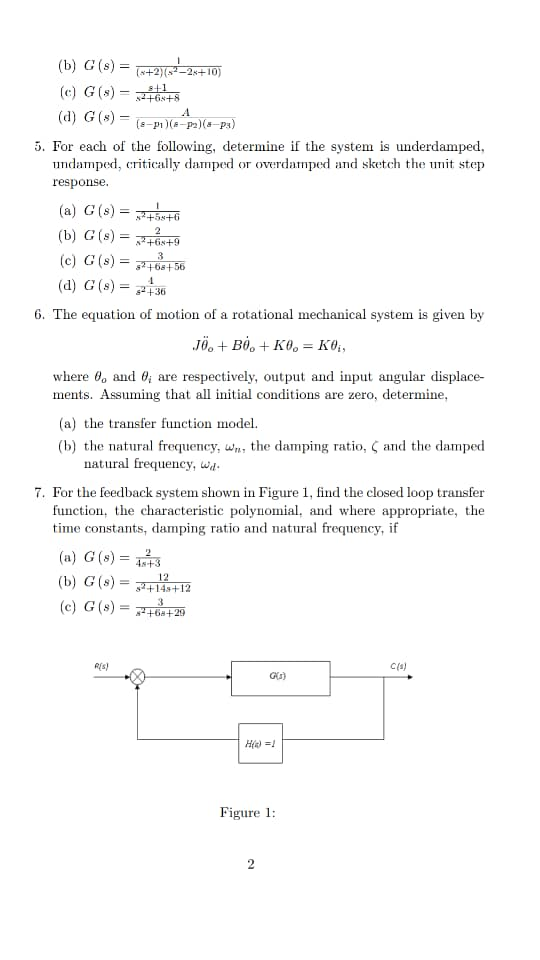5. For each of the following, determine if the system is underdamped, undamped, critically damped or overdamped ad sketch the it step response (a) G (s) = (c) G(s)-t 2+68+ (d) G (s) = 36 6. The equation of motion of a rotational mechanical system is given by where θ° and θί are respectively, output and input angular displace- ments. Assuming that all initial conditions are zero, determine (a) the transfer function model. (b) the natural frequency, w natural frequency,...

• ### Question 5 Using the graphical method (i.e., the method used during the lectures), compute x* h(t),...Question 5 Using the graphical method (i.e., the method used during the lectures), compute x* h(t), where x(t) = e-and h is as shown in the figure. (You must compute x* h, not hx.) For each separate case in your solution, you must state the convolution result and the corresponding range of t as well as show the fully-labelled graph from which this result is derived. Each convolution result may be stated in the form of an integral, but the...

• ### Question 2 Consider the natural sampling applied to a signal, x(t) = sinc"5 The signal is sampled (multiplied) by a periodic (rectangular) pulse train which is shown in Figure (b). Assume the...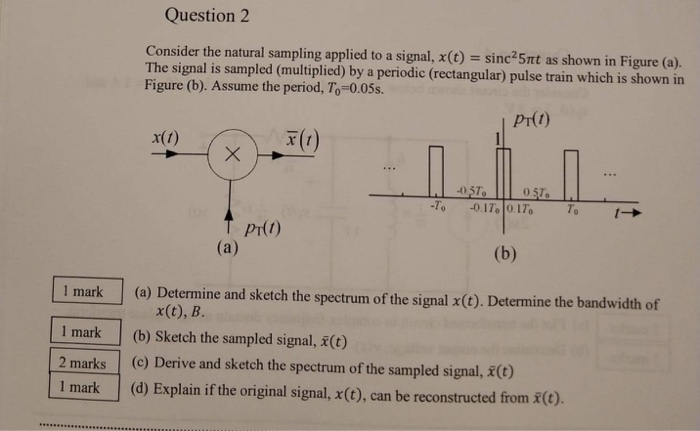Question 2 Consider the natural sampling applied to a signal, x(t) = sinc"5 The signal is sampled (multiplied) by a periodic (rectangular) pulse train which is shown in Figure (b). Assume the period, To-0.05s. πt as shown in Figure (a) Pr(t) x(t) XFO 057 Pt(t) 1 mark (a) Determine and sketch the spectrum of the signal x(t). Determine the bandwidth of x(t), B. 1 mark(b) Sketch the sampled signal, E(t) 2 marks () Derive and sketch the spectrum of the...

Need Online Homework Help?